# Problem 1: Let W = {p(t) € Pz : p'le) = 0}. We know from Problem...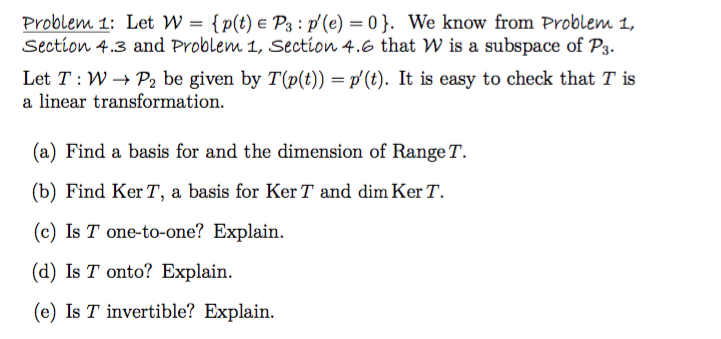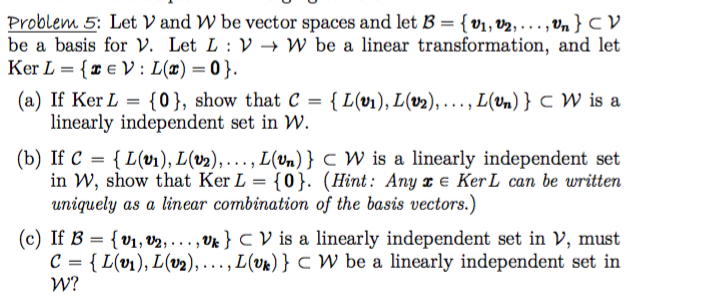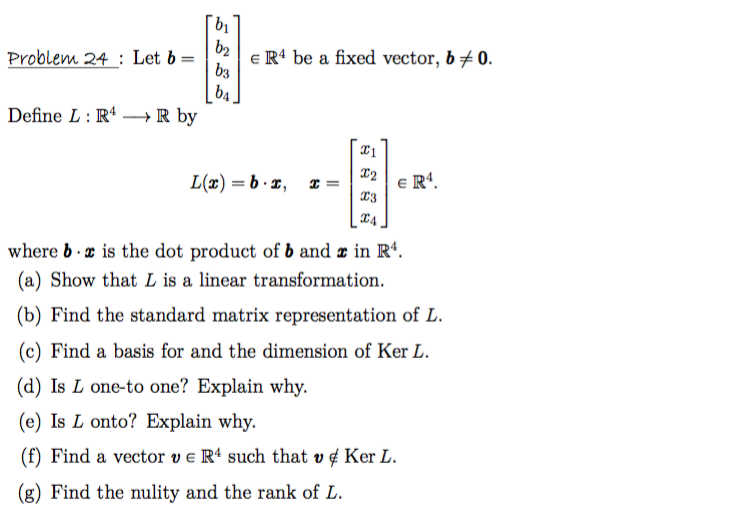Problem 1: Let W = {p(t) € Pz : p'le) = 0}. We know from Problem 1, Section 4.3 and Problem 1, Section 4.6 that W is a subspace of P3. Let T:W+Pbe given by T(p(t)) = p' (t). It is easy to check that T is a linear transformation. (a) Find a basis for and the dimension of Range T. (b) Find Ker T, a basis for Ker T and dim KerT. (c) Is T one-to-one? Explain. (d) Is T onto? Explain. (e) Is T invertible? Explain.
Problem 5: Let V and W be vector spaces and let B = {V1, V2, ..., Un} CV be a basis for V. Let L: V → W be a linear transformation, and let Ker L = {ZEV: L(x)=0}. (a) If Ker L = {0}, show that C = { L(vi), L(02), ..., L(Un) } C W is a linearly independent set in W. (b) If C = {L(vi), L(v2),..., L(Un) } CW is a linearly independent set in W, show that Ker L = {0}. (Hint: Any z e KerL can be written uniquely as a linear combination of the basis vectors.) (c) If B = {V1, V2,..., Uk} CV is a linearly independent set in V, must C = {L(v1), L(v2),..., L(Uk) } CW be a linearly independent set in W?
(bil b2 b3 Problem 24 : Let b = E R4 be a fixed vector, b70. 64 Define L: R4 +R by L(x) = b 2, II 1 22 03 24 ER4 where b.x is the dot product of b and x in R4. (a) Show that L is a linear transformation. (b) Find the standard matrix representation of L. (c) Find a basis for and the dimension of Ker L. (d) Is L one-to one? Explain why. (e) Is L onto? Explain why. (f) Find a vector ve R4 such that v ¢ Ker L. (g) Find the nulity and the rank of L.

For any queries please comment below otherwise, give a thumb's up.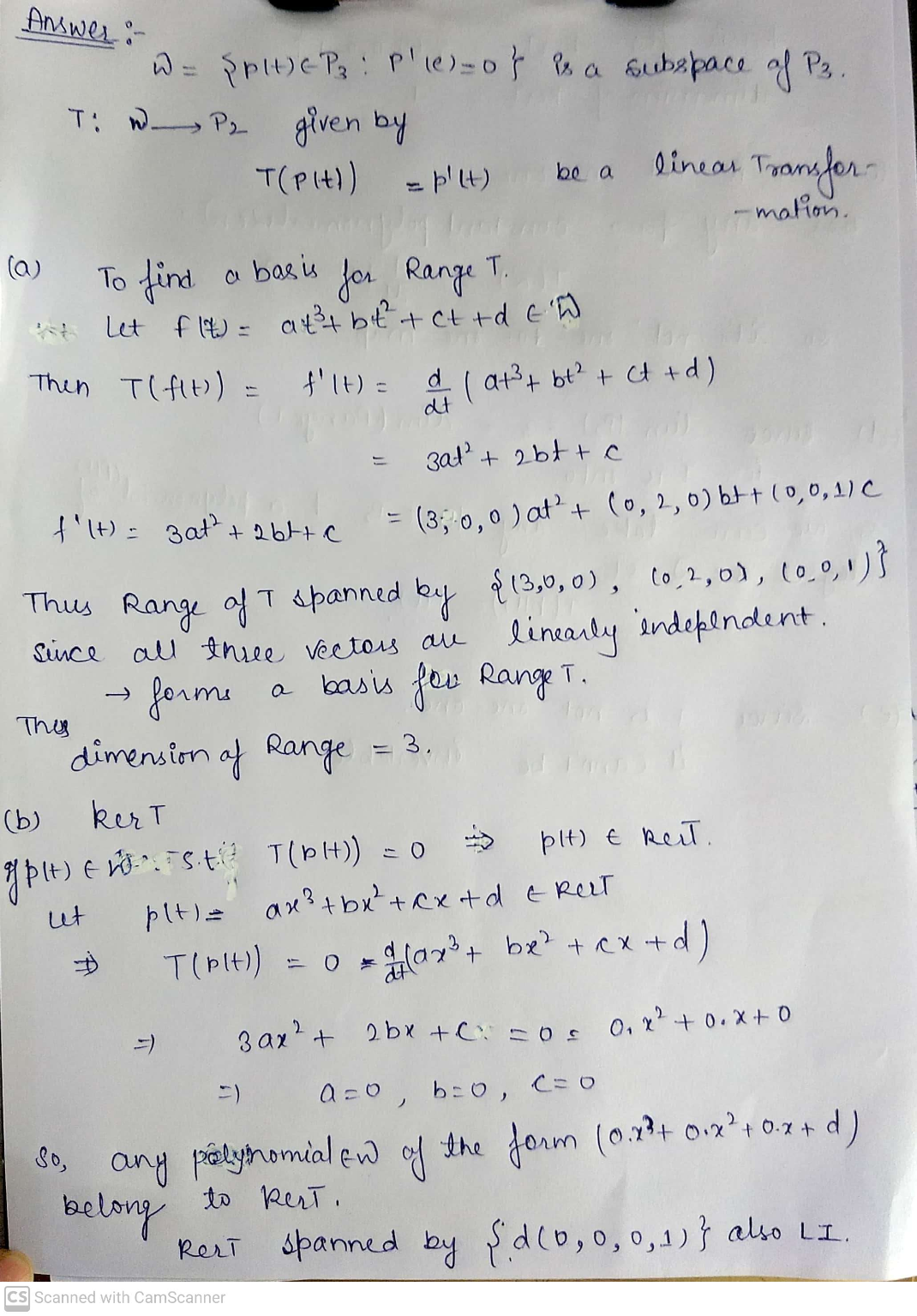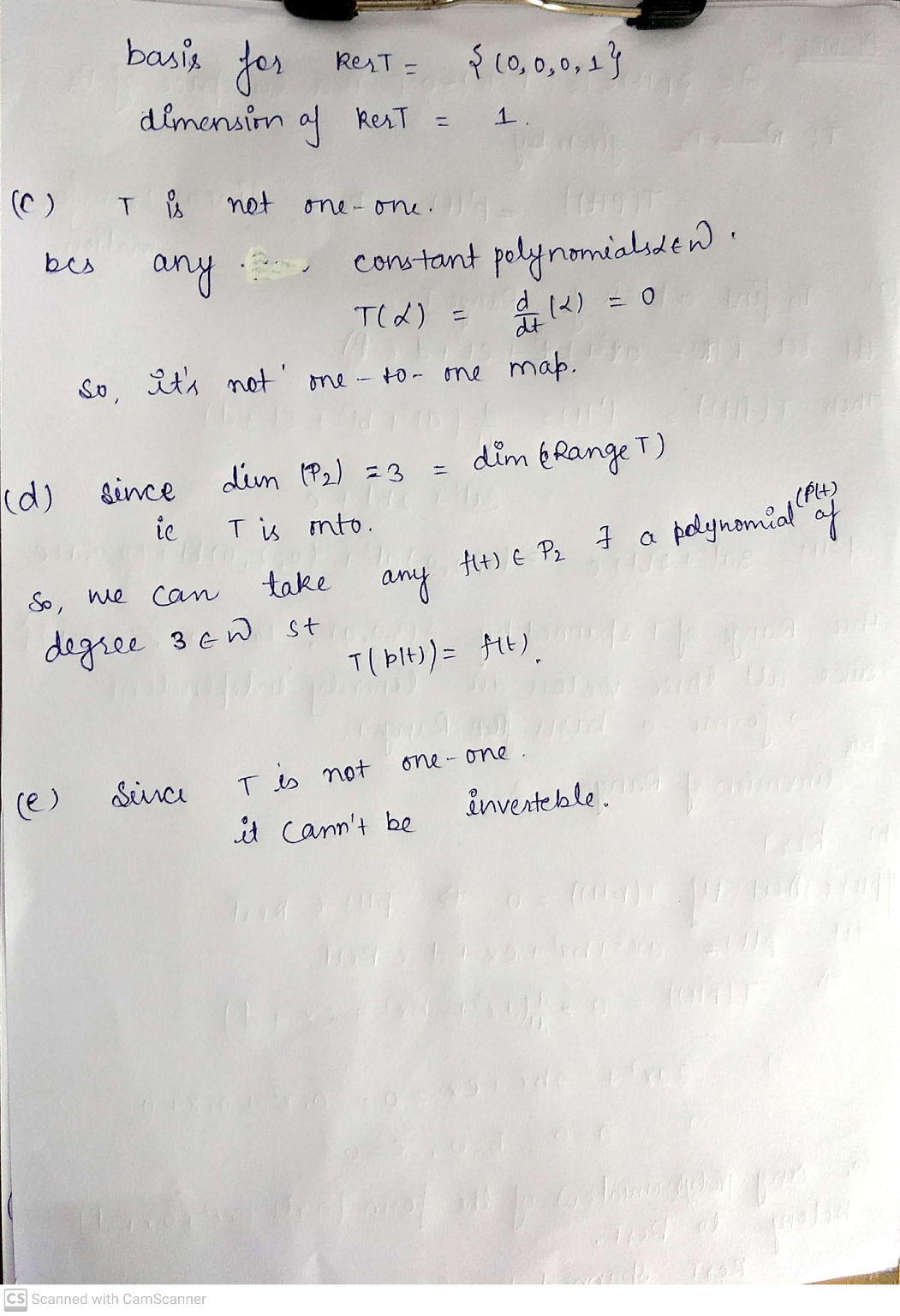##### Add Answer of: Problem 1: Let W = {p(t) € Pz : p'le) = 0}. We know from Problem...
Similar Homework Help Questions
• ### Problem 5: Let V and W be vector spaces and let B = {V1, V2, ...,...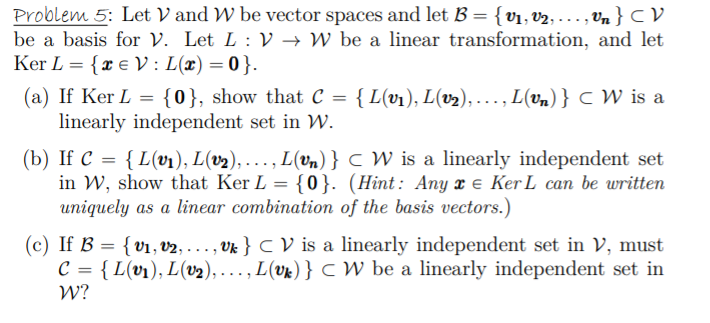Problem 5: Let V and W be vector spaces and let B = {V1, V2, ..., Un} CV be a basis for V. Let L :V + W be a linear transformation, and let Ker L = {2 € V: L(x)=0}. (a) If Ker L = {0}, show that C = {L(v1), L(02), ..., L(vn) } CW is a linearly independent set in W. (b) If C = {L(01), L(V2),..., L(Un)} C W is a linearly independent set in W,...

• ### 7. Let T : V → W be a linear transformation, and let v1,v2,...,vn be vectors...

7. Let T : V → W be a linear transformation, and let v1,v2,...,vn be vectors in V. Suppose that T (v1), T (v2), . . . , T (vn) are linearly independent. Show that v1, v2, . . . , vn are linearly independent.

• ### Please give answer with the details. Thanks a lot! Let T: V-W be a linear transformation between vector spaces V and W...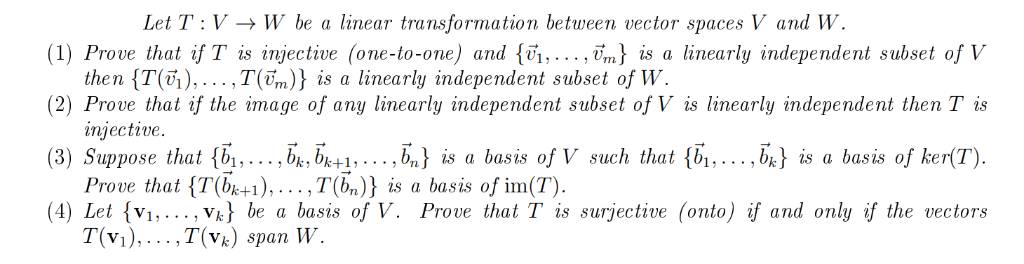Please give answer with the details. Thanks a lot! Let T: V-W be a linear transformation between vector spaces V and W (1) Prove that if T is injective (one-to-one) and {vi,.. ., vm) is a linearly independent subset of V the n {T(6),…,T(ền)} is a linearly independent subset of W (2) Prove that if the image of any linearly independent subset of V is linearly independent then Tis injective. (3) Suppose that {b1,... bkbk+1,. . . ,b,) is a...

• ### Q4. Let T :V + W be a one-to-one linear transformation (i.e. an equation T(u) =...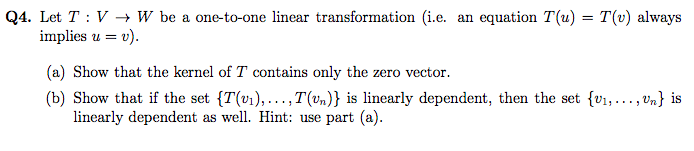Q4. Let T :V + W be a one-to-one linear transformation (i.e. an equation T(u) = T(v) always implies u = v). (a) Show that the kernel of T contains only the zero vector. (b) Show that if the set {T(v1), ...,T(un)} is linearly dependent, then the set {V1, ..., Un} is linearly dependent as well. Hint: use part (a).

• ### 6. Given the points A = (0,0), B = (5,1), C = (2,6) on the plane....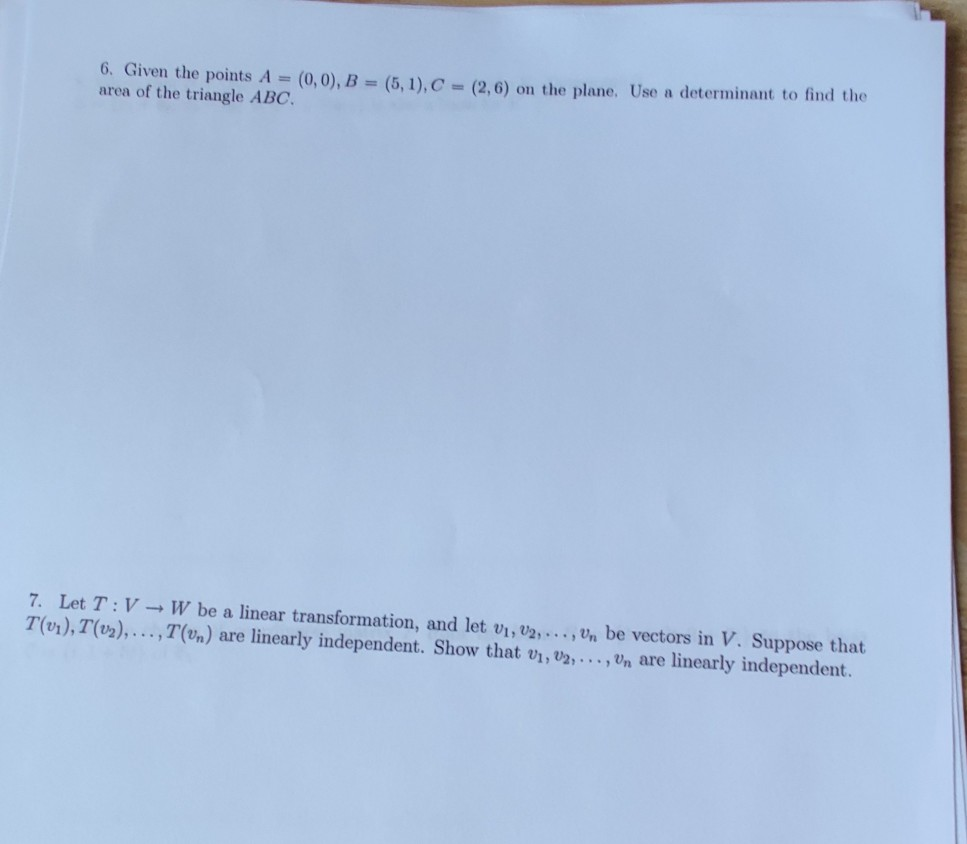6. Given the points A = (0,0), B = (5,1), C = (2,6) on the plane. Use a determinant to find the area of the triangle ABC. 7. Let T:V - W be a linear transformation, and let V1, V2, ..., Un be vectors in V. Suppose that T(vi), T(02),...,T(un) are linearly independent. Show that V1, V2, ..., Vn are linearly independent. 3. Given that 8 - ...) is a basis for a vector space V. Determine if 3 -...

• ### Proble m 3. Let T: V ->W be (1) Prove that if T is then T(),... ,T(Fm)} is a linearly indepen dent subset of W (2) P...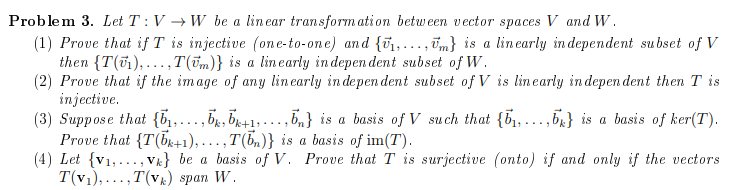Proble m 3. Let T: V ->W be (1) Prove that if T is then T(),... ,T(Fm)} is a linearly indepen dent subset of W (2) Prove that if the image of any linearly in depen dent subset of V is linearly indepen dent then T is injective (3) Suppose that {,... ,b,b^1,...,5} is Prove that T(b1), .. . , T(b,)} is a basis of im(T) (4) Let v1,. Vk} be T(v1),..,T(vk) span W lin ear transform ation between vector...

• ### Q4. Let T :V + W be a one-to-one linear transformation (i.e. an equation T(u) =...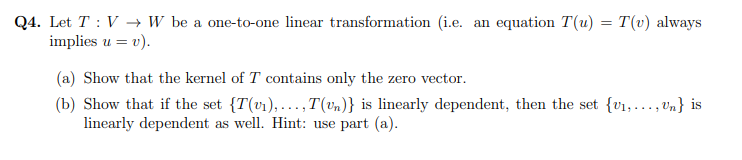Q4. Let T :V + W be a one-to-one linear transformation (i.e. an equation T(u) = T(v) always implies u = v). (a) Show that the kernel of T contains only the zero vector. (b) Show that if the set {T(v1),...,T(Un)} is linearly dependent, then the set {01, ..., Un} is linearly dependent as well. Hint: use part (a).

• ### Q4. Let T :V + W be a one-to-one linear transformation (i.e. an equation T(u) =...Q4. Let T :V + W be a one-to-one linear transformation (i.e. an equation T(u) = T(v) always implies u = v). (a) Show that the kernel of T contains only the zero vector. (b) Show that if the set {T(v1),...,T(Un)} is linearly dependent, then the set {01, ..., Un} is linearly dependent as well. Hint: use part (a).

• ### Q4. Let T :V + W be a one-to-one linear transformation (i.e. an equation T(u) =...Q4. Let T :V + W be a one-to-one linear transformation (i.e. an equation T(u) = T(v) always implies u = v). (a) Show that the kernel of T contains only the zero vector. (b) Show that if the set {T(v1),...,T(Un)} is linearly dependent, then the set {01, ..., Un} is linearly dependent as well. Hint: use part (a).

• ### Q4. Let T :V + W be a one-to-one linear transformation (i.e. an equation T(u) =...Q4. Let T :V + W be a one-to-one linear transformation (i.e. an equation T(u) = T(v) always implies u = v). (a) Show that the kernel of T contains only the zero vector. (b) Show that if the set {T(v1),...,T(Un)} is linearly dependent, then the set {01, ..., Un} is linearly dependent as well. Hint: use part (a).

Free Homework App